Information for developers, analysts and administrators

# Using AGGR instead of IF for complex dimensions

In this post, I’ll focus on a quick tip that encourages the use of Aggr for complex dimensions.

What’s a complex dimension? Most of the time, we’ll construct the database for our app so that our users can simply drag or select a field for a dimension and use it—that’s a simple dimension. Sometimes, however, we’ll need to use a formula for a dimension. This usually occurs when we need to apply filtering logic to the dimension, format the dimension (for example, a date format), or create a custom string for a dimension—these are examples of complex dimensions, and depending upon the situation, they can get very complex.

When you select a simple dimension in a chart or table, Qlik Sense will show the selection you made in the filter bar. For example, I we selected the value “US” for the dimension Region, we’d see something like this…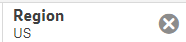But what about a complex dimension? When you use a formula as a dimension, Qlik Sense does a good job of figuring out how to determine what actual fields (dimensions) are being filtered, but in many situations, it can’t. In those cases, instead of seeing one or more dimensions in the filter bar, you’ll see a formula. While technically correct, this can be confusing, especially to end users.

Here’s an example.

I made a simple table that displays information for film actors and actresses who have been nominated for academy awards. I’m using a simple dimension “Display Name” for the table.I’ll select a group of names.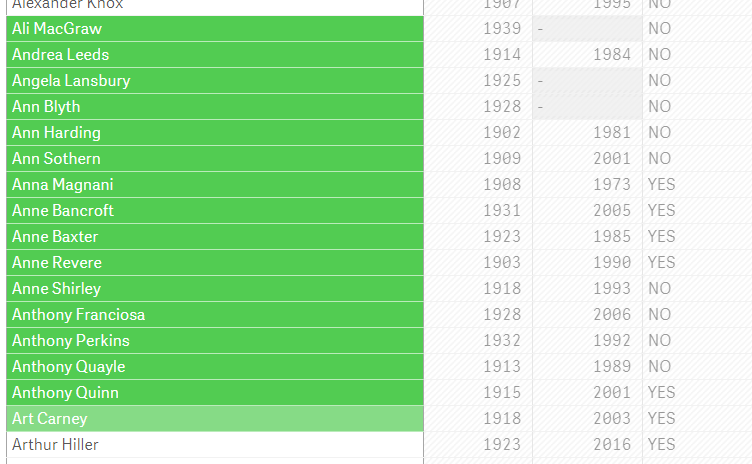We’ll see the dimension name and number of selections displayed in the filter bar at the top of the screen.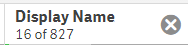Now let’s look at an example of a more complex dimension. I want to change the “Name” column to show formatted text comprised of the Display Name and whether the person is an actor or actress. I’ll change the dimension to use the formula below.

=[Display Name] & If(Gender = 'M', ' (actor)', If(Gender = 'F', ' (actress)'))

Here is the result.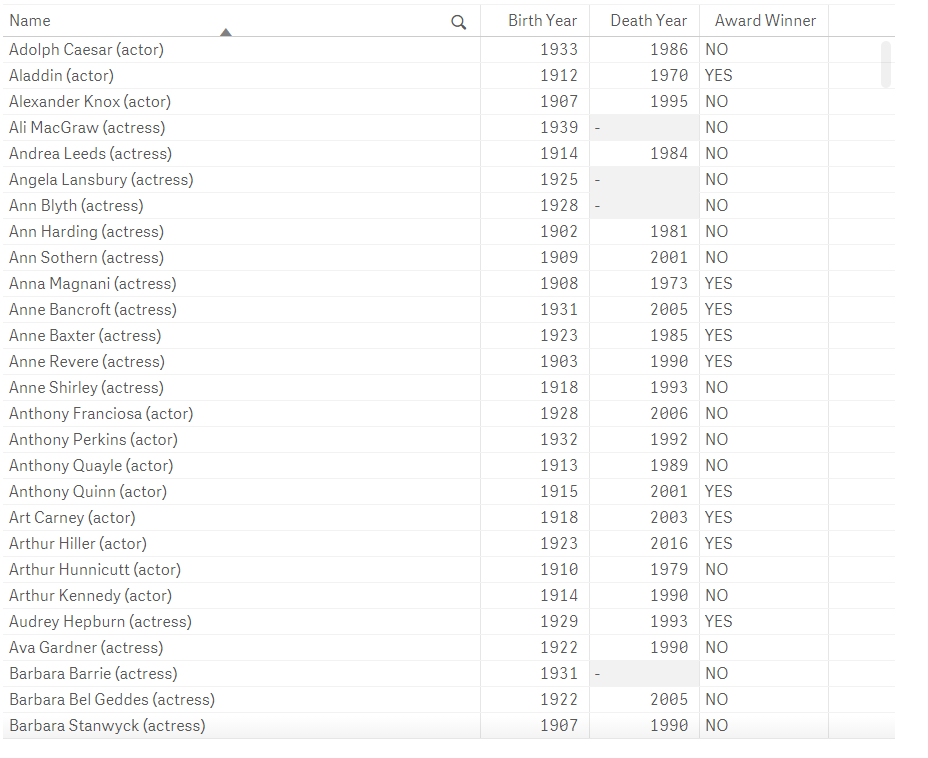Let’s make the same selection that we did earlier.Notice what has happened in the filter bar (below). Qlik Sense displays the formula we used instead of the dimension “Display Name”. If you hover over it, it will actually display the entire expression that was used.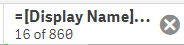We actually did select those Display Names and if the dimension appears in a Filter Pane object, it will correctly show this. But, the information shown in the filter bar at the top of the screen is confusing, because it doesn’t really show this.

Fortunately, we have another option. Let’s change the formula to use an AGGR function. The resulting expression will look like this.

=Aggr([Display Name] & If(Gender = 'M', ' (actor)', If(Gender = 'F', ' (actress)')), [Display Name])

This might look confusing at first, but all I’ve done is use the same expression as the expression in the Aggr function and used the dimension as the grouping dimension in the Aggr. This produces the exact same set of dimension values and my table will look exactly the same.

If I make the same Name selection again the filter bar will now show the dimension name “Display Name” instead of the expression.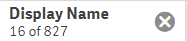This is exactlywhat we want and will work in almost all cases, regardless of how complex my Aggr statement is. Using Aggr is a flexible way to ensure that your calculated dimension will correctly display in the filter bar.

If you’d like to understand more about what you can do with Aggr, there are many examples on QlikCommunity and in the Qlik documentation.